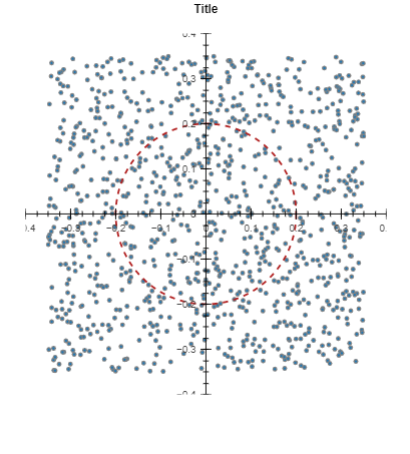# Tick Labels being cut-off

Hello,

I am getting started with Bokeh to automate plot generation but I am stuck with the tick labels not being shown.

I tried playing around with the plot dimensions and also the frame dimensions.
None of my approaches work.

How can I set the range of both axis with the labels being shown (0.4 and -0.4 in this case).

Code below thanks

``````def single_orb_plot(acc_x, acc_y, title, radius):

p = figure(toolbar_location= None, outline_line_color = None,
title= title + "\n")

#Set Dimensions
p.frame_width = 400
p.frame_height = 400
p.plot_width = 500
p.plot_height = 500
p.min_border = 50
#     p.match_aspect = True

# Set Title
p.title.text_color = "black"
p.title.text_font = "arial"
p.title.align = "center"

# Set Axis Locations & Style
p.axis.fixed_location = 0
p.axis.axis_line_color = 'black'
p.axis.ticker = tickers.BasicTicker(num_minor_ticks = 4)
p.x_range = ranges.Range1d(start = -0.4, end = 0.4)
p.y_range = ranges.Range1d(start = -0.4, end = 0.4)

# Ticks Length
p.axis.major_tick_in = 6
p.axis.major_tick_out = 6
p.axis.minor_tick_in = 3
p.axis.minor_tick_out = 3

# Gridlines
p.grid.grid_line_color = None

p.line(x = acc_x, y = acc_y,line_width = 1.5, line_color = '#828282')
p.circle(x=0, y=0, color="navy",line_color = "firebrick",
line_width=2, line_dash = 'dashed', fill_color = None,